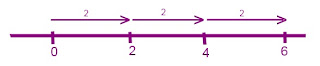## Monday, March 7, 2011

### Hanna's Great Big Book of Integers

Chapter 1

Integers are positive and negative numbers. Usually, integers are represented by the colours red and blue, red symbolizing positive numbers and blue symbolizing negative numbers. You can show integers by using number lines and integer chips.

A zero pair is a set of numbers opposite of each other.
Ex. +6, -6
+10, -10

+43, -43

If you add them together, they become zero.

Ex. -8 + 8 = 0

A saying that can help you when it comes to subtracting integers is "when subtracting something that isn't there use a zero pair".

Multiplying Integers

(+2) x (+3) = 6
(-2) x (+3) = -6
(-2) x (-3) = 6

Sign Rule (negative signs)
Even - When you have an even number of negative factors the product is positive
Odd - When you have an odd number of negative factors the product is positive

Chapter 3

Dividing Integers

Partitive division is finding out how many groups it can fit in

6 ÷ 2 = 3-6 ÷ (-2) =3
Quotative division is when you share an amount with groups.

(-6) ÷ 2 = -3
Rule for Division - When there is one or an odd number of negative signs the quotient is negative

6 ÷ 2 = 3
The answer is positive because there are no negative signs

-6 ÷ (-2) =3
The answer is positive because there is an even amount of negative signs
(-6) ÷ 2 = -3
The answer is negative since there is an odd number of negative signs
6 ÷ (-2) = -3
The answer is negative because there is an odd amount of negative signs

Chapter 4
Order of Operations with Integers

(+5) x (-3) + (-6) ÷ (+3) =
[(+5) x (-3)] + [(-6) ÷ (+3)] =
(-15) + (-2) = -17